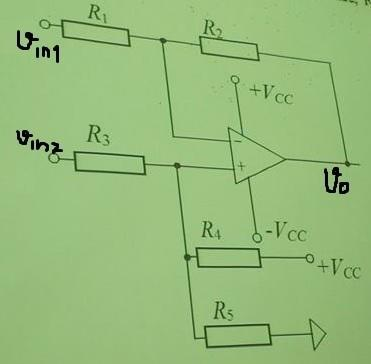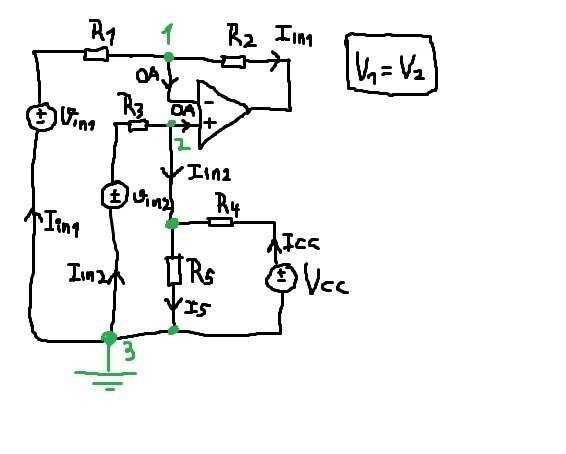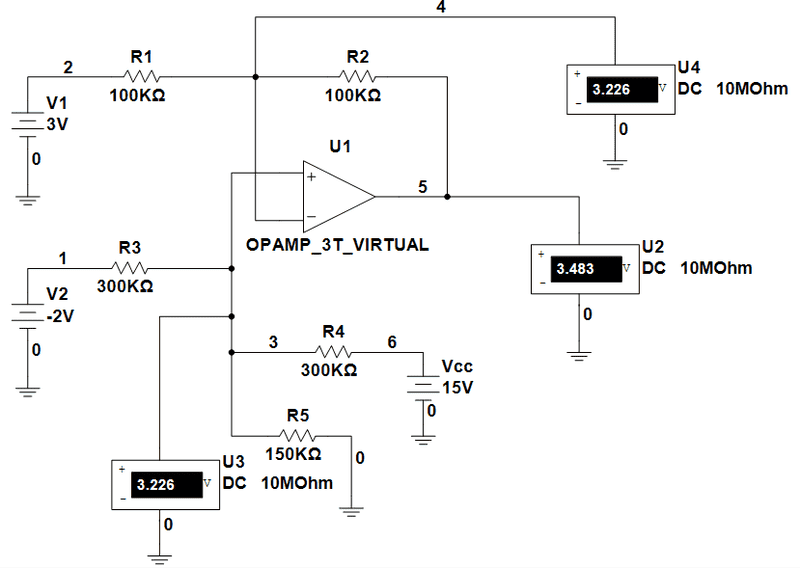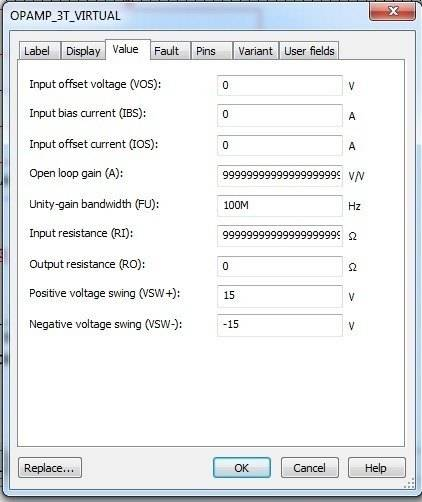# OPAMP Circuit problem

• Engineering
Hi!
Find output voltage Vo if Vin1=3V, Vin2=-2V, R1=R2=R3=100KΩ, R4=300KΩ, R5=150KΩ, VCC=15V.Applying KCL at node 2 we get:
$$-Iin2+I5-Icc=0$$
$$-\frac{V3-V2+Vin2}{R3}+\frac{V2-V3}{R5}-\frac{V3-V2+Vcc}{R4}=0$$
$$-\frac{Vin2-V2}{R3}+\frac{V2}{R5}-\frac{Vcc-V2}{R4}=0$$
$$V1=V2=\frac{Vin2/R3+Vcc/R4}{1/R3+1/R5+1/R4}=1.5V$$
But Multisim give me different voltage V2:As you can see, Multisim measure 3.226V.
Here is OPAMP setup (since it's ideal, I put some huge numbers for A and input resistance, output resistance is 0):Any idea what's wrong?

Last edited:

gneill
Mentor
In your Multisim circuit you have set R3 to 300k, but the problem statement says it is 100k.

•1 person
I can't figure out how I didn't see it :) Thanks!

gneill
Mentor
I can't figure out how I didn't see it :) Thanks!

Sometimes a fresh pair of eyes on the problem can pick out the things you miss repeatedly due to familiarity!

You might want to take note that in your simulation the "voltmeters" have an impedance of 10MΩ, and will influence the circuit behavior to some extent (it'll show up in the second or third digit of the values I'm guessing). So don't be surprised if your calculated values are almost but not quite the same as your "measured" ones!